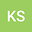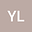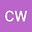On approximate solutions for convex semi-infinite programming with uncertainty
•••• Ke Su,
• Yumeng Lin,
• Chen Wang
Ke Su
Hebei University

Corresponding Author:3558325465@qq.com

Author ProfileYumeng Lin
Hebei University
Author ProfileChen Wang
Hebei University
Author Profile## Abstract

In this paper, we consider approximate solutions (also called $\varepsilon$-solutions) for semi-infinite optimization problems that objective function and constraint functions with uncertainty data are all convex, and establish robust counterpart of convex semi-infinite program and then consider approximate solutions for its. Moreover, the robust necessary condition and robust sufficient theorems are obtained. Then the duality results of the Lagrangian dual approximate solution is given by the robust optimization approach under a cone constraint qualification.